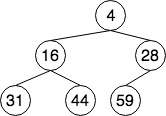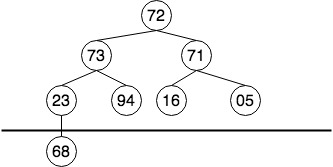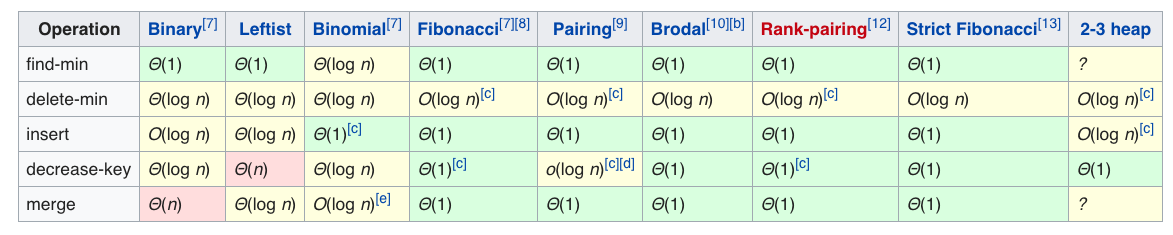# 堆

1. $K_1<=K_{2i+1}$
2. $K_1<=K_{2i+2}$1. 建堆，将一组无序的数组织成堆的形式
• 思路1：将n数组成的无序数组进行原地建堆操作
• 思路2：将n个无序的数一个一个进行插入操作
2. 新元素入堆后如何调整堆
• 将其放到数组最右边的位置
• 递归进行SiftUp调整
3. 堆顶元素出堆后如何调整
• 将数组最后一个节点的值付给堆顶元素
• 删除最后一个元素
• 堆顶节点递归进行SiftDown调整

### 堆的表示

class Heap{
vector<int> heap;
int n; //堆大小
int count; //堆中元素个数

public:
Heap(int capicity){
heap = vector<int>(capacity, 0);
n = capacity;
count = 0;
}
Heap(vector<int>& arr){
capacity = arr.size();
count = capacity;
heap = arr;
buildHeap(heap);
}
void buildHeap(vector<int>& arr);
void push(int data);
int top(){ return heap; };
void pop();
private:
int left_child_index(int pos){
int index = pos*2+1;
return index >= n?-1:index;
}
int right_child_index(int pos){
int index = pos*2+2;
return index?=n?-1:index;
}
int parent_index(int pos){
return (pos-1)/2;
}
void sift_up(size_t pos);
void sift_down(size_t pos);
};


### SiftDown调整

//递归调整
void sift_down(vector<int>& heap, size_t position){
//找到左右节点的index
size_t l = left_child_index(position);
size_t r = right_child_index(position);

//待删除节点为叶子节点
if(l == -1 && r == -1){
return;
}
//待删除节点左子节点为空，说明当前已经是叶子节点
else if(l == -1 ){
return;
}
//待删除节点的右子节点为空，比较左节点
else if(r == -1 ){
if(heap[i] > heap[l]){
swap(heap[i], heap[l]);
sift_down(l);
}
}else{
//待删除节点的左右子节点都不空，找到最小的
size_t index = heap[l] < heap[r] ? l:r;
if(heap[i] > heap[index]){
swap(heap[i],heap[index]);
sift_down(index);
}
}
}


### SiftUp调整

void sift_up(vector<int>& heap, size_t pos){
if(pos == 0){
return;
}
int p = parent_index(pos);
if(heap[pos]<heap[p]){
//交换父子节点
swap(heap[pos], [p]);
//递归调整
sift_up(p);
}
}


### 建堆

1. n个关键码放到一维数组中，整体不是最小堆
2. 由完全二叉树的特性，有一半的节点⌊n/2⌋是叶子节点，它们不参与建堆的过程
• i≥⌊n/2⌋ 时，以关键码Ki为根的子树已经是堆
3. 从倒数第二层最右边的非叶子节点开始（完全二叉树数组i=⌊n/2-1⌋的元素），依次向前，进行递归SiftDown调整。1. 按照完全二叉树排布，形成树形结构，如上图
2. 成树后，可以看到有4个节点(⌊4/2⌋)已经是叶子节点，它们不需要参与建堆的过程
3. 23开始（数组第i=⌊4/2-1⌋=3项）依次进行sift_down调整，顺序依次是:23,71 ,73 ,72
void buildHeap(vector<int>& heap){
for (int i=count/2-1; i>=0; i--)
sift_down(heap,i);
}
}


1. $n$个节点的堆，高度为$d=⌊\log_2^{n}+1⌋$，设根为第$0$层，第$i$层节点数为$2^i$
2. 考虑一个元素在队中向下移动的距离
• 大约一半的节点深度为$d-1$，不移动（叶）。
• 四分之一的节点深度为$d-2$，而它们至多能向下移动一层。
• 树中每向上一层，节点的数目为前一层的一半，而子树高度加一。
• 因此元素移动的最大距离的总数为：

### 插入元素

void push(vector<>int data){
if(count >= n){
return ; //堆满了
}
++count;
heap[count] = data;
//递归调整
sift_up(heap,count);
}


### 删除元素

void pop(){
if(count == 0){
return ; //堆中没有数据
}
heap = heap[count]; //交换堆顶和末尾元素
heap.pop_back(); //删除末尾元素
--count ;
//堆顶元素sift_down
sift_down(heap,1);
}


## 堆的其它实现## 堆排序

void heap_sort(vector<int>& a){
buildHeap(a);
int k = a.size()-1;
while(k>=0){
swap(a,a[k]);
--k;
sift_down(a,0);
}
}


## 堆的相关应用

• 求一个无序数组中第K大的元素

class Solution {
public:
int findKthLargest(vector<int>& nums, int k) {
priority_queue<int,std::vector<int>,std::greater<int>> pq;
for(auto x:nums){
if(pq.size()<k){
pq.push(x);
}else{
if(x>pq.top()){
pq.pop();
pq.push(x);
}
}
}
return pq.top();
}
};


• 无序数组中位数问题

int findMedium(vector<int>& arr){
priority_queue<int> maxHeap;
priority_queue<int,std::vector<int>,std::greater<int>> minHeap;

for(auto x : arr){
if(maxHeap.empty() || x < maxHeap.top()){
maxHeap.push(x);
}else{
minHeap.push(x);
}
if(minHeap.size() > maxHeap.size()){
maxHeap.push(minHeap.top());
minHeap.pop();
}
if(maxHeap.size() - minHeap.size() > 1){
minHeap.push(maxHeap.top());
maxHeap.pop();
}
}
return maxHeap.top();
}